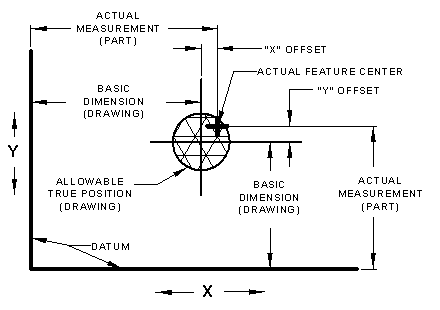#### True Position GD&T Tolerance Calculator

Calculators | Technology Store

(This calculator requires a java - enabled browser)

This calculator calculates position tolerances utilizing principles and concepts withinASME Y14.5-2009 and ASME Y14.5M - 1994, Geometric Dimensioning and Tolerancing (GD&T).

For the equations behind this calculator, see:
"Geometric Boundaries"
Interpretation and Application of
Geometric Dimensioning and TolerancingNotes:

1. Units may be given as inches, mm, meters or whatever.
2. Units DO NEED to be consistant (e.g. all inches or all mm).
3. NAN = Values out of range for entered values (values not possible) .

 GD&T True Position - Measured Offset Calculator Offset (X) Offset (Y) Calculated True Position Specified True Position (+) Excess Tolerance (-) Tolerance Deficit

GD&T True Position Calculator With Basic Dimension Data

Notes:

1. Units may be given as inches, mm, meters or whatever.
2. Units DO NEED to be consistant (e.g. all inches or all mm).
 Basic Dimension X Axis = Actual Measured X Axis = Offsett X = Basic Dimension Y = Actual Measured Y Axis = Offsett Y = Drawing True Position Specified = Calculated Radius Offset = Calculated True Position = Tolerance Excess(+)Tolerance Deficit (-)

Related Resources:© Copyright 2000 - 2021, by Engineers Edge, LLC www.engineersedge.com
All rights reserved
Disclaimer | Feedback | Advertising | Contact

Date/Time: